# Java Script HTML DOM Java vs Java Script

• Slides: 35Java. Script, HTML, DOMJava vs Java. Script • "When Java applets failed, Java. Script became the 'Language of the Web' by default. [. . . ] It is unfortunate that Java failed [as a language for web browsers]" • "Java. Script is the only language that is found in all browsers. [. . . ] Java. Script is flourishing" • "In Java. Script, there is a beautiful, elegant, highly expressive language that is buried under a steaming pile of good intentions and blunders. [. . . It is] one of the most popular languages [. . . and] one of the most despised" • Source: Douglas Crockford, "Java. Script: The Good Parts", 2008, O'Reilly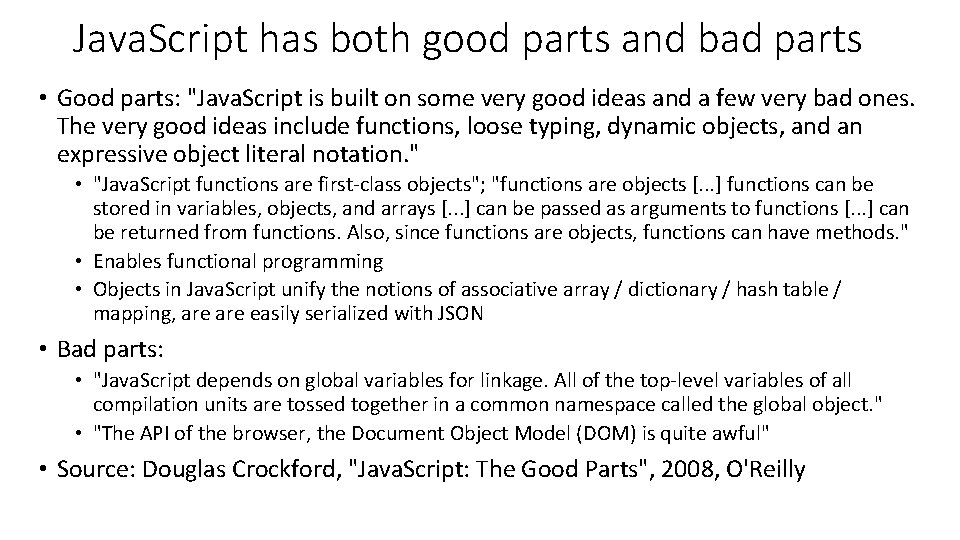Java. Script has both good parts and bad parts • Good parts: "Java. Script is built on some very good ideas and a few very bad ones. The very good ideas include functions, loose typing, dynamic objects, and an expressive object literal notation. " • "Java. Script functions are first-class objects"; "functions are objects [. . . ] functions can be stored in variables, objects, and arrays [. . . ] can be passed as arguments to functions [. . . ] can be returned from functions. Also, since functions are objects, functions can have methods. " • Enables functional programming • Objects in Java. Script unify the notions of associative array / dictionary / hash table / mapping, are easily serialized with JSON • Bad parts: • "Java. Script depends on global variables for linkage. All of the top-level variables of all compilation units are tossed together in a common namespace called the global object. " • "The API of the browser, the Document Object Model (DOM) is quite awful" • Source: Douglas Crockford, "Java. Script: The Good Parts", 2008, O'Reilly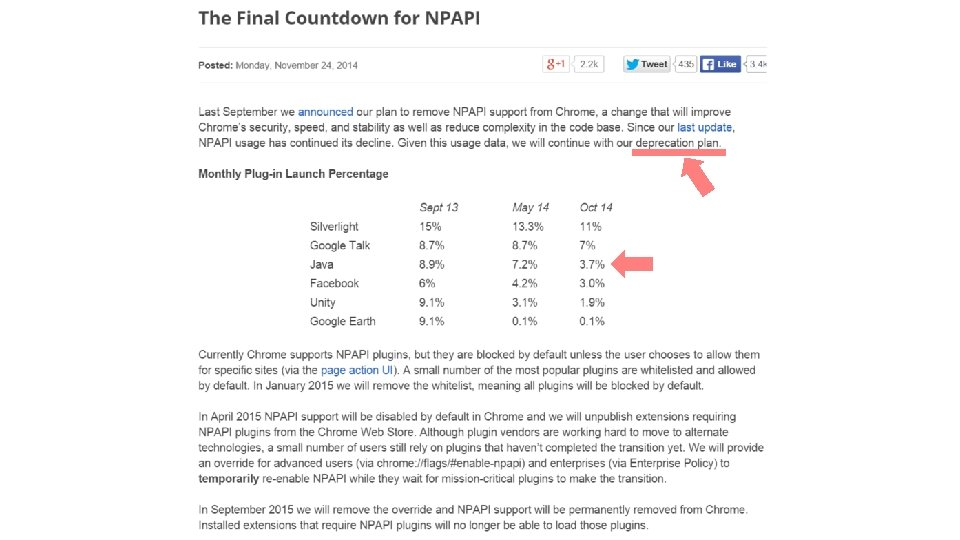Java. Script: the language (ECMAScript 5, supported by Chrome 23+, IE 10+, Fire. Fox 21+, Opera 15+, Safari 6+, i. OS Safari 7+) http: //kangax. github. io/compat-table/es 5/ (For a bit of history about ECMAScript 4 and Adobe Action. Script 3: http: //whydoeseverythingsuck. com/2008/08/ru-roh-adobe-screwed-byecmascript. html )// boolean var flag = true; // implicit type flag = false; // string var fruit. Name = "apple"; // use ". . . fruit. Name = 'grape'; //. . . or ' as quotes. fruit. Name = 'or' + "ange"; // Use + to concatenate. // The === sign compares the content of strings, not references: fruit. Name === "orang" + "e" // true fruit. Name. length === 6 // true // Special characters: ", ', \, n (newline), r (carriage return), t (tab) // Hexadecimal unicode: "u 0041" === "A", // "u 2661" === "♡", 'u. D 83 Du. DCA 9' === "�� " Remember!// number: always stored in double precision floating point: 64 bits 1. 0 === 1 // true var x = 3; // x === 3; x = 3. 14; // x === 3. 14 x = 355/113; // x === 3. 1415929203539825 Math. floor(x) === 3 // integer part x = 1/137; // approximates the fine structure constant // x === 0. 00729927005 x = 1/0; // x === Infinity x=0/0; // x === Na. N Remember!Conversion between strings and numbers: var x = 32; x. to. String() === "32" x. to. String(16) === "20" // base 16 parse. Int("20") === 20 parse. Int("20", 16) === 32 The second parameter for parse. Int() is the base, which is 10 by default. It is recommended that this base always be explicitly specified, otherwise, a string starting with zero might be interpreted in base 8 (octal), which is risky when working with dates. parse. Int("09") === 0 // before ECMAScript 5 parse. Int("09") === 9 // ECMAScript 5 parse. Int("0 x 100") === 256 // base 16, because the string starts with 0 x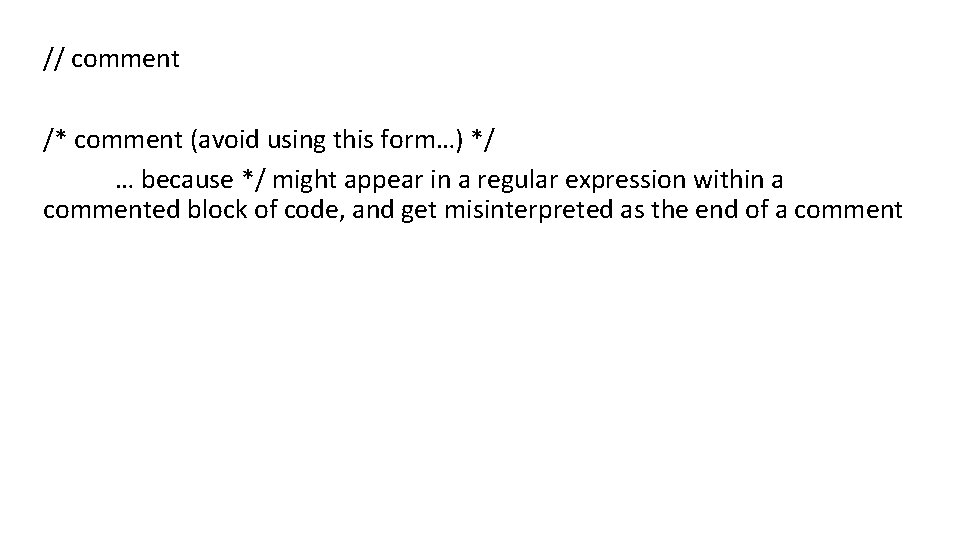// comment /* comment (avoid using this form…) */ … because */ might appear in a regular expression within a commented block of code, and get misinterpreted as the end of a comment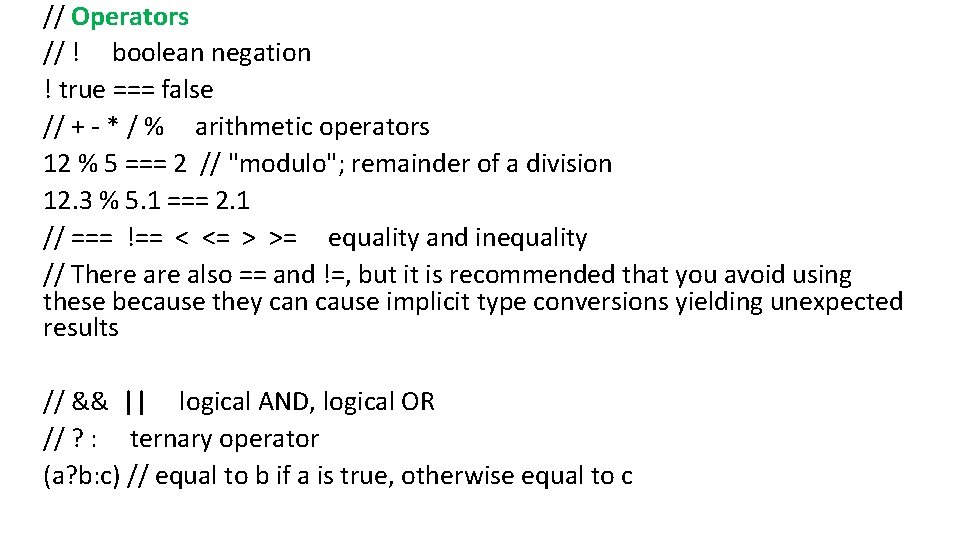// Operators // ! boolean negation ! true === false // + - * / % arithmetic operators 12 % 5 === 2 // "modulo"; remainder of a division 12. 3 % 5. 1 === 2. 1 // === !== < <= > >= equality and inequality // There also == and !=, but it is recommended that you avoid using these because they can cause implicit type conversions yielding unexpected results // && || logical AND, logical OR // ? : ternary operator (a? b: c) // equal to b if a is true, otherwise equal to c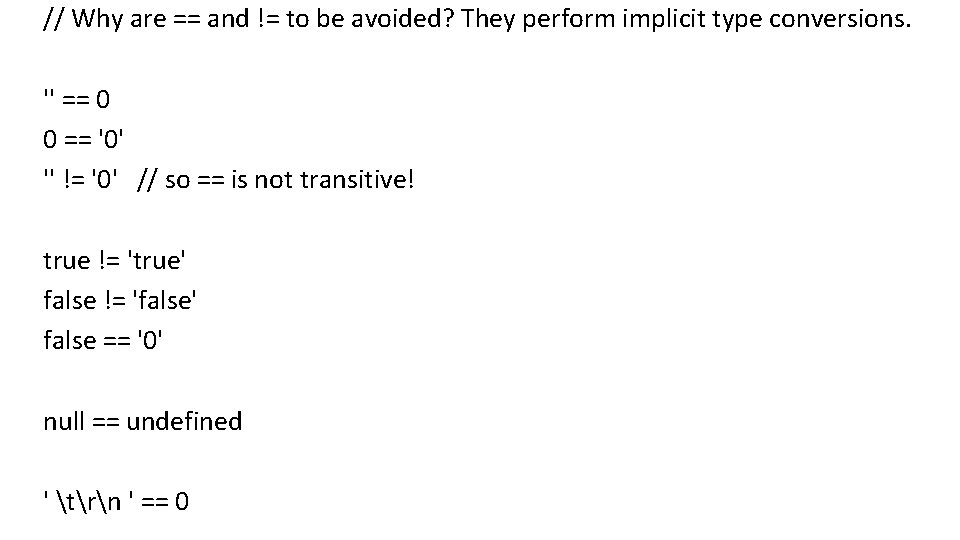// Why are == and != to be avoided? They perform implicit type conversions. '' == 0 0 == '0' '' != '0' // so == is not transitive! true != 'true' false != 'false' false == '0' null == undefined ' trn ' == 0// Be careful with implicit type conversions '2'+'1' === "21" // string + string === string '2'-'1' === 1 // string - string === number '2'+1 === "21" // string + number === string '2'-1 === 1 // string - number === number '2' + + '1' === "21" 'foo' + + 'bar' === "foo. Na. N" '2' + - '1' === "2 -1" '2' + - - + + - + - - - '-1' === "21" var x = 3; '2' + x - x === 20 '2' - x + x === 2if ( expression ) {. . . } else {. . . } Remember!switch ( expression ) { case a: . . . break; case b: . . . break; default: . . . }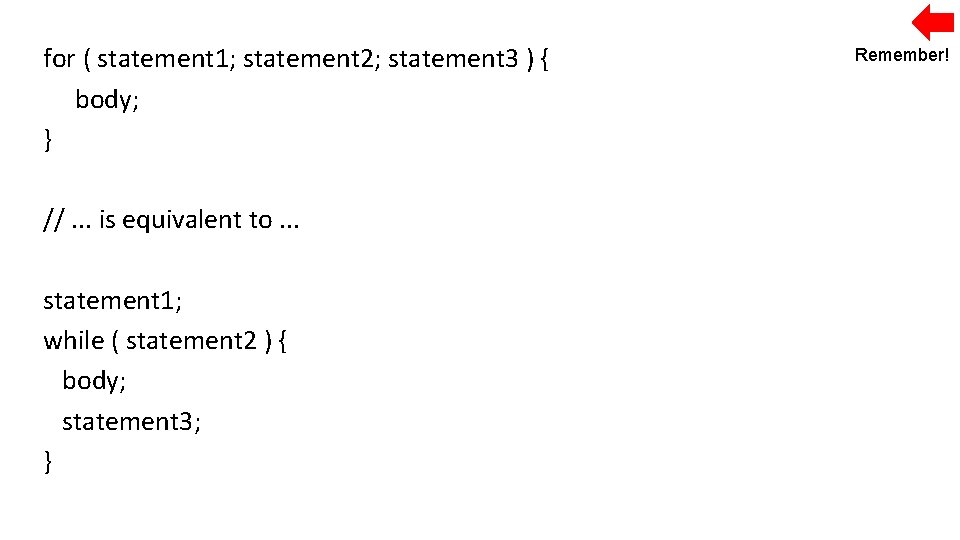for ( statement 1; statement 2; statement 3 ) { body; } //. . . is equivalent to. . . statement 1; while ( statement 2 ) { body; statement 3; } Remember!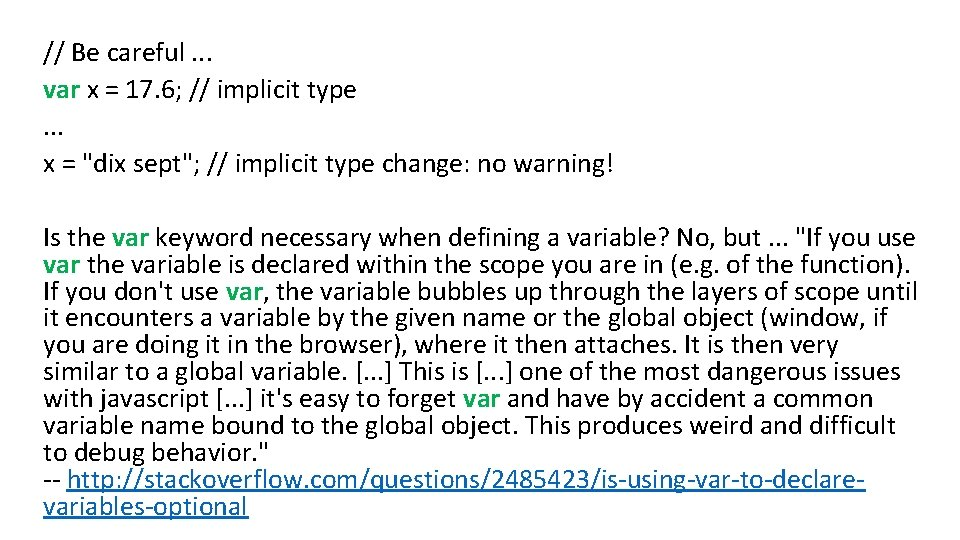// Be careful. . . var x = 17. 6; // implicit type. . . x = "dix sept"; // implicit type change: no warning! Is the var keyword necessary when defining a variable? No, but. . . "If you use var the variable is declared within the scope you are in (e. g. of the function). If you don't use var, the variable bubbles up through the layers of scope until it encounters a variable by the given name or the global object (window, if you are doing it in the browser), where it then attaches. It is then very similar to a global variable. [. . . ] This is [. . . ] one of the most dangerous issues with javascript [. . . ] it's easy to forget var and have by accident a common variable name bound to the global object. This produces weird and difficult to debug behavior. " -- http: //stackoverflow. com/questions/2485423/is-using-var-to-declarevariables-optional// Object property continent 1 = { name Remember! name : "North America", population : 530000000, // 530 million value area : 24709000 // in km 2 }; continent 1. name === "North America" // like a Java object continent 1["name"] === "North America" // like a dictionary // We can dynamically add properties continent 1. population. Density = continent 1. population / continent 1. area; continent 1. population. Density === 21. 449674207778543 // We can remove properties delete continent 1. area; continent 1. area === undefined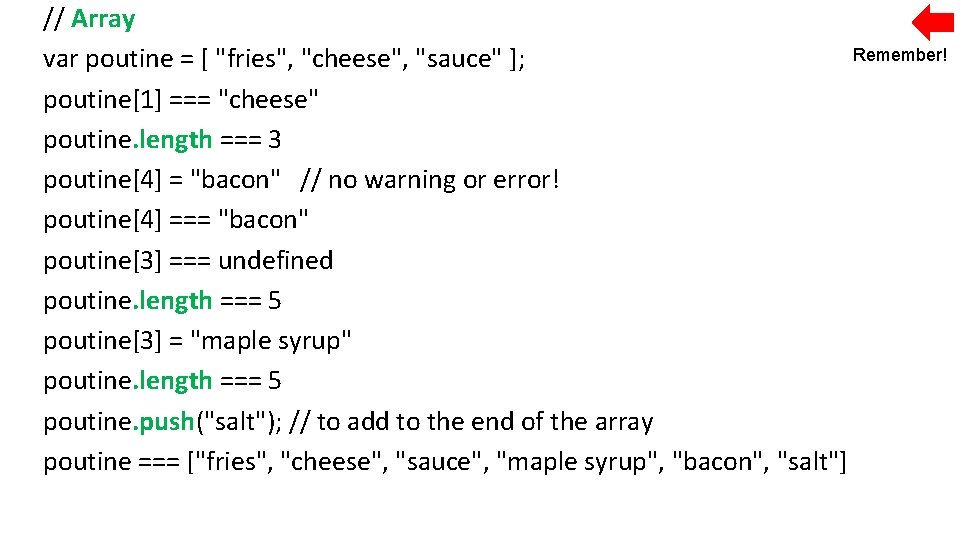// Array Remember! var poutine = [ "fries", "cheese", "sauce" ]; poutine === "cheese" poutine. length === 3 poutine = "bacon" // no warning or error! poutine === "bacon" poutine === undefined poutine. length === 5 poutine = "maple syrup" poutine. length === 5 poutine. push("salt"); // to add to the end of the array poutine === ["fries", "cheese", "sauce", "maple syrup", "bacon", "salt"]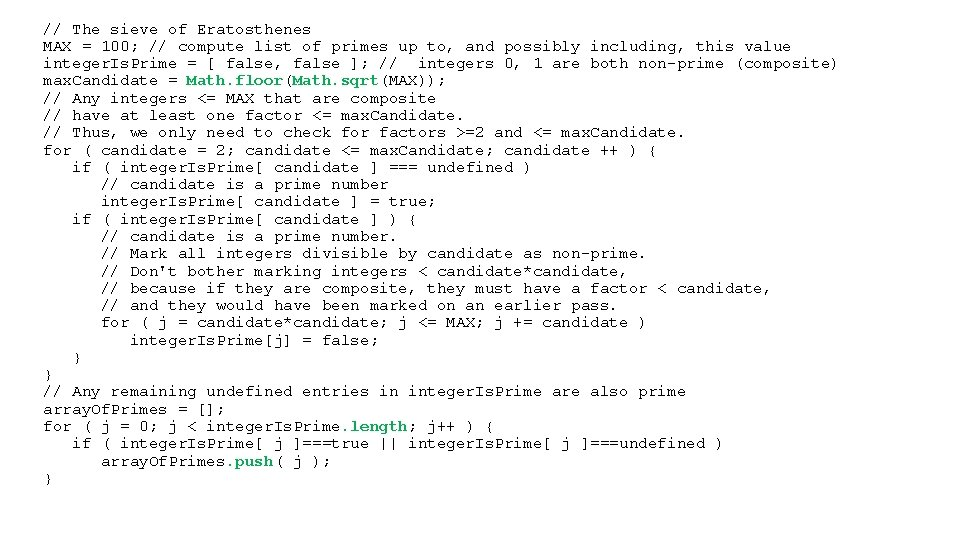// The sieve of Eratosthenes MAX = 100; // compute list of primes up to, and possibly including, this value integer. Is. Prime = [ false, false ]; // integers 0, 1 are both non-prime (composite) max. Candidate = Math. floor(Math. sqrt(MAX)); // Any integers <= MAX that are composite // have at least one factor <= max. Candidate. // Thus, we only need to check for factors >=2 and <= max. Candidate. for ( candidate = 2; candidate <= max. Candidate; candidate ++ ) { if ( integer. Is. Prime[ candidate ] === undefined ) // candidate is a prime number integer. Is. Prime[ candidate ] = true; if ( integer. Is. Prime[ candidate ] ) { // candidate is a prime number. // Mark all integers divisible by candidate as non-prime. // Don't bother marking integers < candidate*candidate, // because if they are composite, they must have a factor < candidate, // and they would have been marked on an earlier pass. for ( j = candidate*candidate; j <= MAX; j += candidate ) integer. Is. Prime[j] = false; } } // Any remaining undefined entries in integer. Is. Prime are also prime array. Of. Primes = []; for ( j = 0; j < integer. Is. Prime. length; j++ ) { if ( integer. Is. Prime[ j ]===true || integer. Is. Prime[ j ]===undefined ) array. Of. Primes. push( j ); }Remember! // Array (continued) poutine === ["fries", "cheese", "sauce", "maple syrup", "bacon", "salt"] sub. Array = poutine. splice(2, 1); // starting index, and how many to extract sub. Array === ["sauce"] poutine === ["fries", "cheese", "maple syrup", "bacon", "salt"] sub. Array = poutine. splice(1, 3); sub. Array === ["cheese", "maple syrup", "bacon"] poutine === ["fries", "salt"] // Use. shift() instead of. splice(0, 1) to remove the first element. // An array may contain a mix of types! var jumble = [ 2, true, "salad", { x: 100, y: 200 } , [3, 4] ]; jumble. x === 100 jumble === 3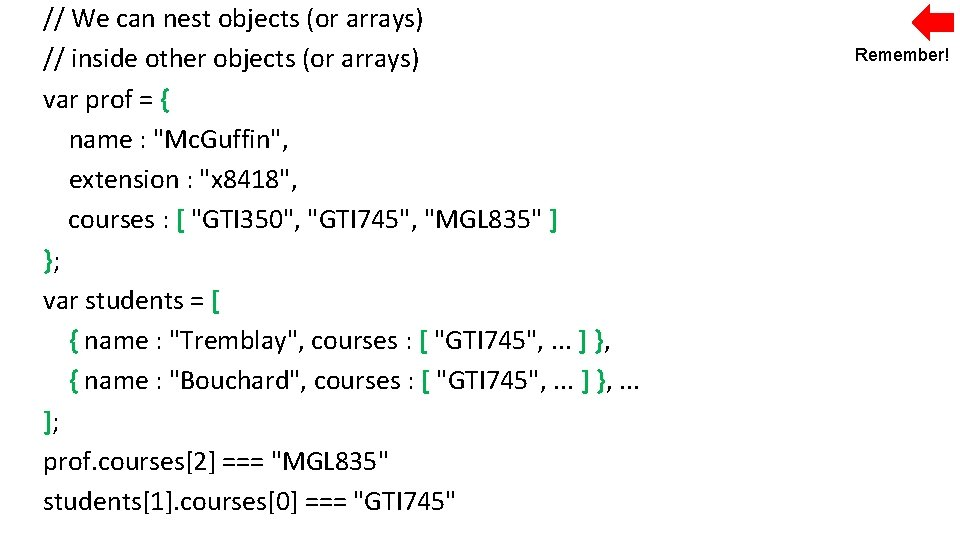// We can nest objects (or arrays) // inside other objects (or arrays) var prof = { name : "Mc. Guffin", extension : "x 8418", courses : [ "GTI 350", "GTI 745", "MGL 835" ] }; var students = [ { name : "Tremblay", courses : [ "GTI 745", . . . ] }, { name : "Bouchard", courses : [ "GTI 745", . . . ] }, . . . ]; prof. courses === "MGL 835" students. courses === "GTI 745" Remember!// A for in loop on an object var prof = { name : "Mc. Guffin", extension : "x 8418", courses : [ "GTI 350", "GTI 745", "MGL 835" ] }; for ( var j in prof ) { // j is a string containing the name of a property in prof. // There is no guarantee that we will visit the properties // in the same order that they were defined! console. log( "prof. " + j + " === " + prof[ j ] + " and j is a " + typeof(j) ); } // output: prof. name === Mc. Guffin and j is a string prof. extension === x 8418 and j is a string prof. courses === GTI 350, GTI 745, MGL 835 and j is a string// A for in loop on an array var courses = [ "GTI 350", "GTI 745", "MGL 835" ]; for ( var j in courses ) { // j is a string containing the index of an element in courses. // There is no guarantee that we will visit the elements // in ascending order! console. log( "courses[" + j + "] === " + courses[ j ] + " and j is a " + typeof(j) ); } // output: courses === GTI 350 and j is a string courses === GTI 745 and j is a string courses === MGL 835 and j is a string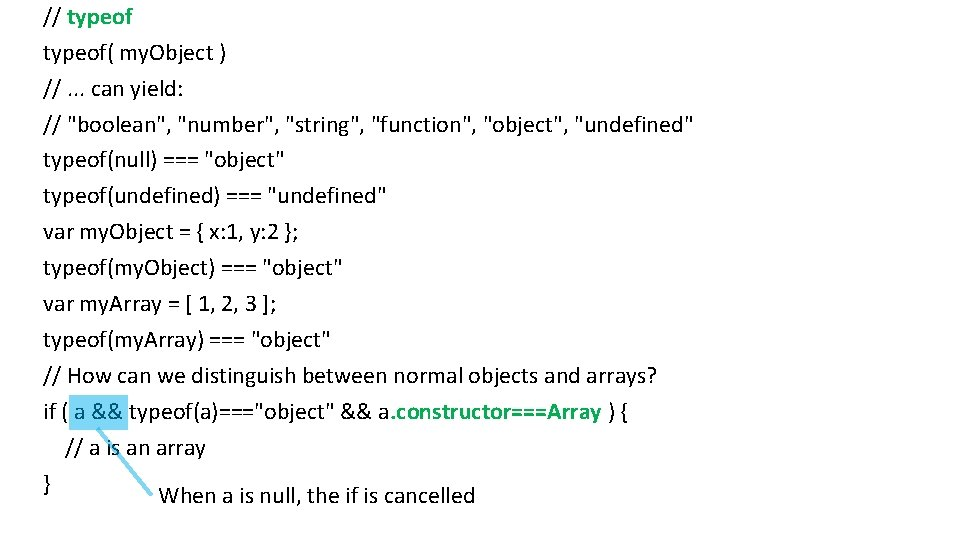// typeof( my. Object ) //. . . can yield: // "boolean", "number", "string", "function", "object", "undefined" typeof(null) === "object" typeof(undefined) === "undefined" var my. Object = { x: 1, y: 2 }; typeof(my. Object) === "object" var my. Array = [ 1, 2, 3 ]; typeof(my. Array) === "object" // How can we distinguish between normal objects and arrays? if ( a && typeof(a)==="object" && a. constructor===Array ) { // a is an array } When a is null, the if is cancelled// Function var my. Two. Norm = function two. Norm( x, y, z ) { return Math. sqrt( x*x + y*y + z*z ); } // two. Norm is the name of the function. If this name is not given, // the function is anonymous. This name is useful for doing recursion. // my. Two. Norm is a variable storing the function. var result = my. Two. Norm(1, 2, 3); // 3. 7416573867739413 // Even if the caller doesn’t provide enough arguments, or too many arguments, // the call still executes var result = my. Two. Norm(1, 2); // z is undefined // result === Na. N// Function (continued) // For each call, the function receives two implicit parameters: // this (an object), and arguments (an array with a. length). // There a few ways to call a function: // 1. "function invocation pattern" my. Two. Norm(1, 2, 3); // this is the global object (In other words, when the code inside the function uses this, it will be referring to the global object, which is window in the case of code inside a web browser) // 2. "apply invocation pattern" (apply() is a method that is automatically defined on all functions) my. Two. Norm. apply( my. Obj, [1, 2, 3] ); // this is my. Obj // It’s as if my. Two. Norm were a method on my. Obj. Using this invocation pattern, we can effectively call a function as if it were a method on any object!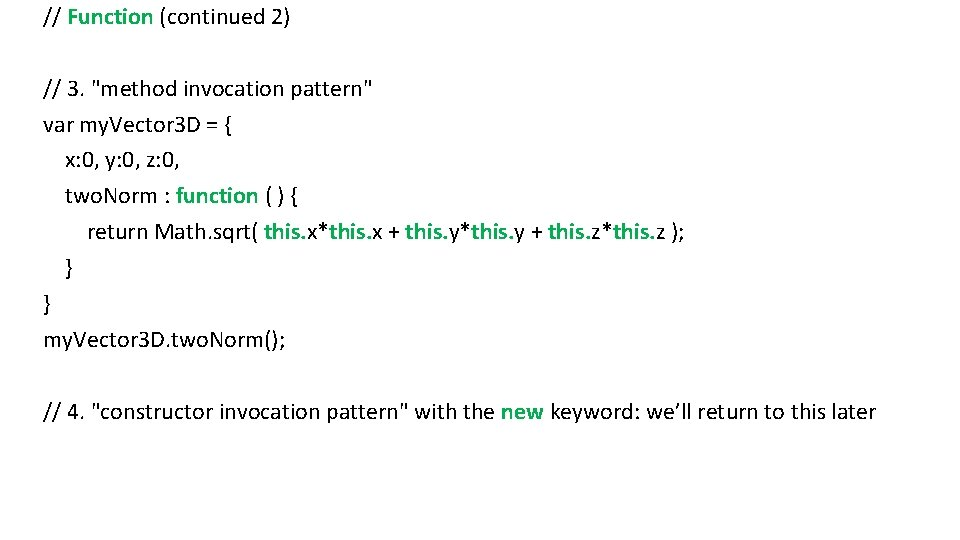// Function (continued 2) // 3. "method invocation pattern" var my. Vector 3 D = { x: 0, y: 0, z: 0, two. Norm : function ( ) { return Math. sqrt( this. x*this. x + this. y*this. y + this. z*this. z ); } } my. Vector 3 D. two. Norm(); // 4. "constructor invocation pattern" with the new keyword: we’ll return to this later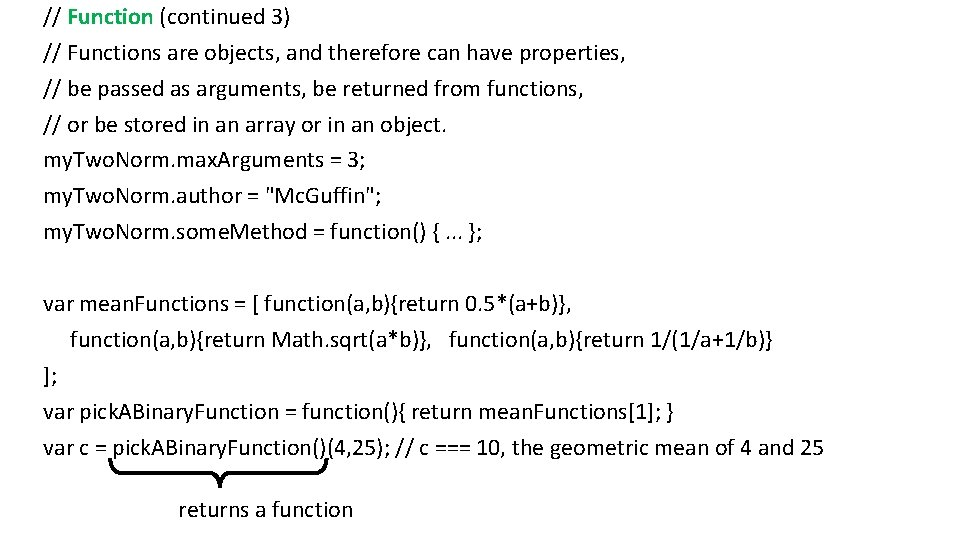// Function (continued 3) // Functions are objects, and therefore can have properties, // be passed as arguments, be returned from functions, // or be stored in an array or in an object. my. Two. Norm. max. Arguments = 3; my. Two. Norm. author = "Mc. Guffin"; my. Two. Norm. some. Method = function() {. . . }; var mean. Functions = [ function(a, b){return 0. 5*(a+b)}, function(a, b){return Math. sqrt(a*b)}, function(a, b){return 1/(1/a+1/b)} ]; var pick. ABinary. Function = function(){ return mean. Functions; } var c = pick. ABinary. Function()(4, 25); // c === 10, the geometric mean of 4 and 25 returns a function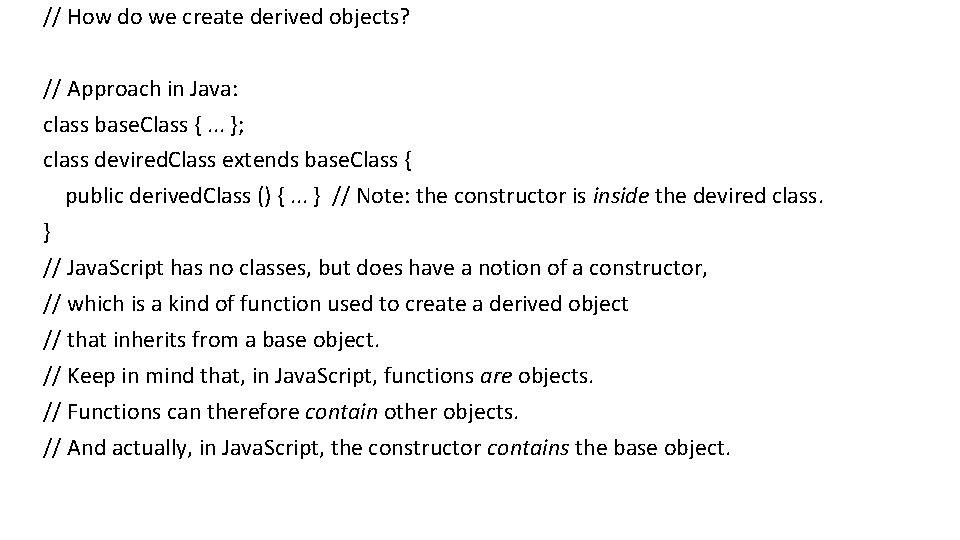// How do we create derived objects? // Approach in Java: class base. Class {. . . }; class devired. Class extends base. Class { public derived. Class () {. . . } // Note: the constructor is inside the devired class. } // Java. Script has no classes, but does have a notion of a constructor, // which is a kind of function used to create a derived object // that inherits from a base object. // Keep in mind that, in Java. Script, functions are objects. // Functions can therefore contain other objects. // And actually, in Java. Script, the constructor contains the base object.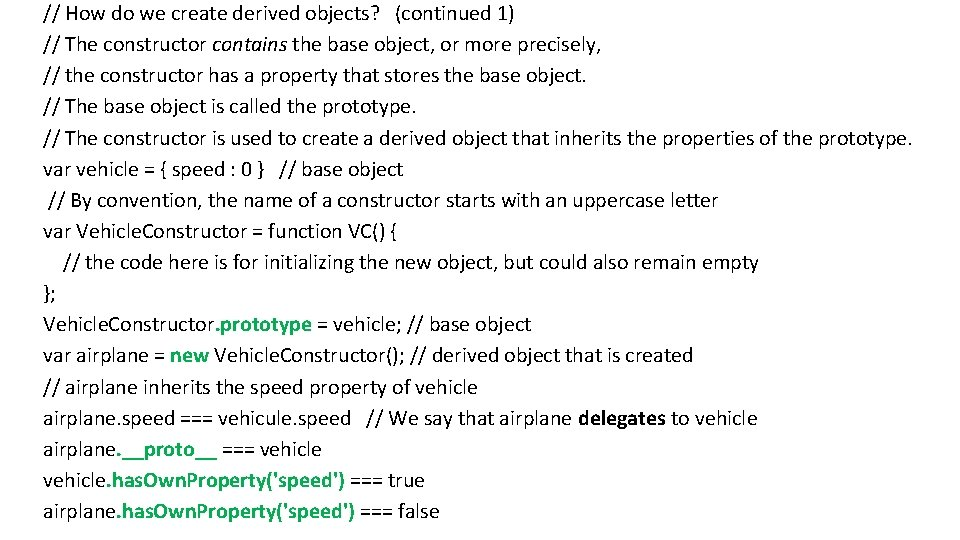// How do we create derived objects? (continued 1) // The constructor contains the base object, or more precisely, // the constructor has a property that stores the base object. // The base object is called the prototype. // The constructor is used to create a derived object that inherits the properties of the prototype. var vehicle = { speed : 0 } // base object // By convention, the name of a constructor starts with an uppercase letter var Vehicle. Constructor = function VC() { // the code here is for initializing the new object, but could also remain empty }; Vehicle. Constructor. prototype = vehicle; // base object var airplane = new Vehicle. Constructor(); // derived object that is created // airplane inherits the speed property of vehicle airplane. speed === vehicule. speed // We say that airplane delegates to vehicle airplane. __proto__ === vehicle. has. Own. Property('speed') === true airplane. has. Own. Property('speed') === false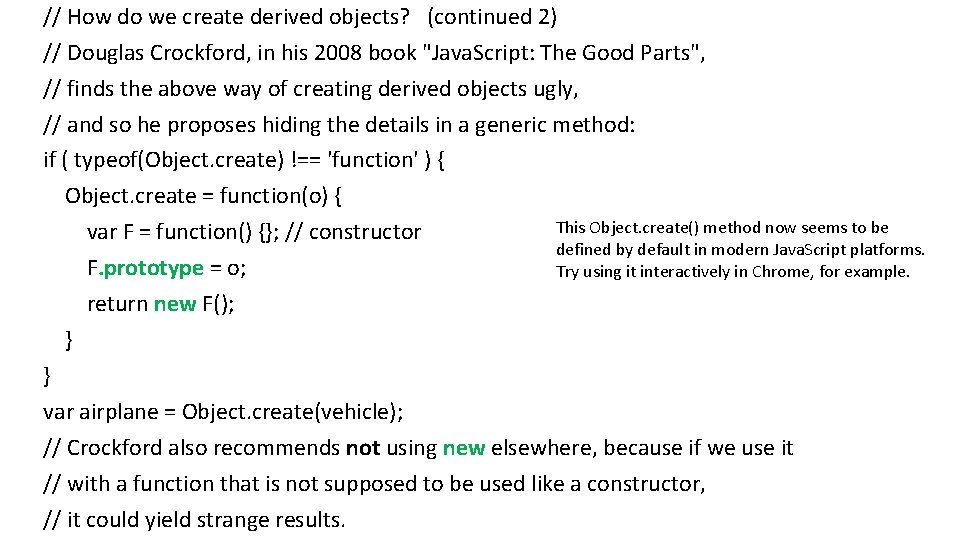// How do we create derived objects? (continued 2) // Douglas Crockford, in his 2008 book "Java. Script: The Good Parts", // finds the above way of creating derived objects ugly, // and so he proposes hiding the details in a generic method: if ( typeof(Object. create) !== 'function' ) { Object. create = function(o) { This Object. create() method now seems to be var F = function() {}; // constructor defined by default in modern Java. Script platforms. F. prototype = o; Try using it interactively in Chrome, for example. return new F(); } } var airplane = Object. create(vehicle); // Crockford also recommends not using new elsewhere, because if we use it // with a function that is not supposed to be used like a constructor, // it could yield strange results.// How do we create derived objects? (continued 3) More information on derived objects: http: //javascript. crockford. com/prototypal. html http: //kevinoncode. blogspot. ca/2011/04/understanding-javascript-inheritance. html http: //pivotallabs. com/javascript-constructors-prototypes-and-the-new-keyword/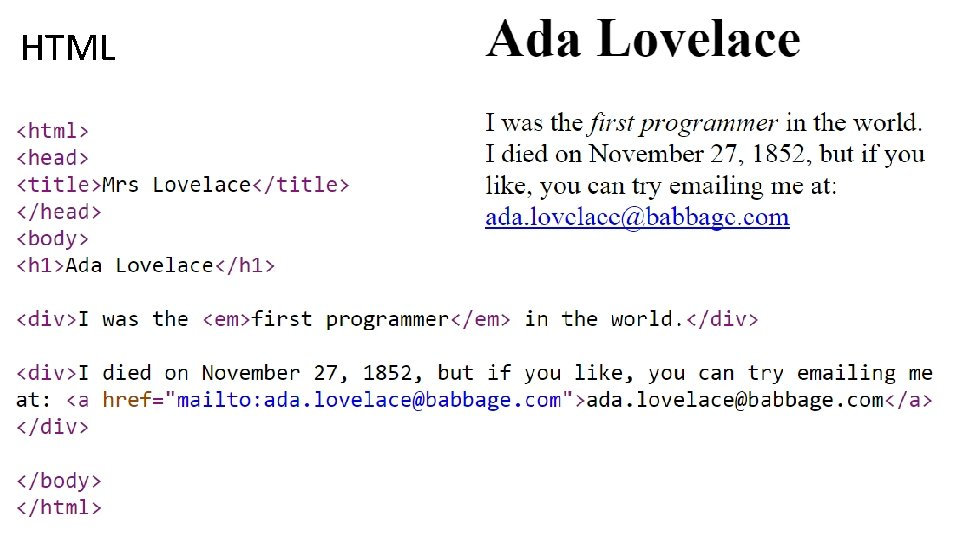HTML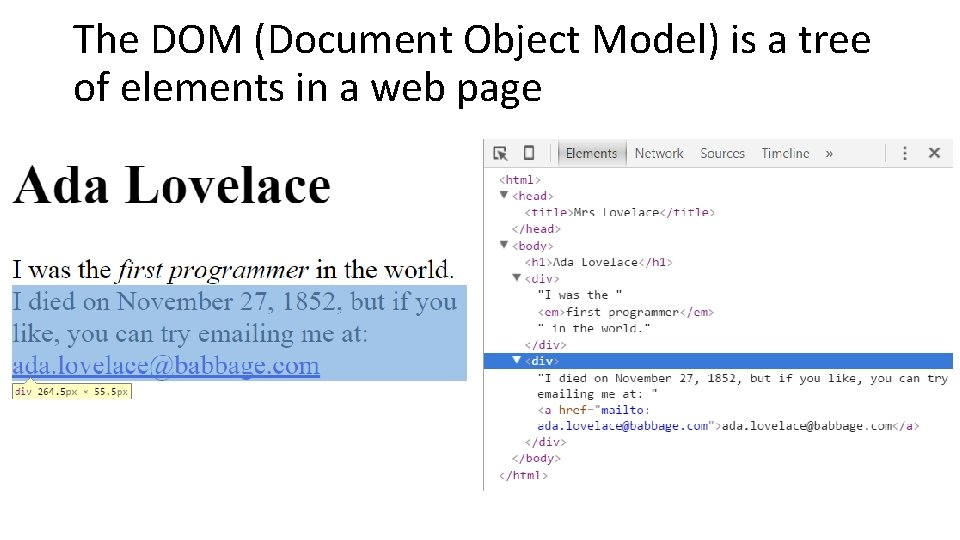The DOM (Document Object Model) is a tree of elements in a web pageHTML + Java. Script This example uses Java. Script to protect the email address from spammers that use web crawlers to harvest email addresses.